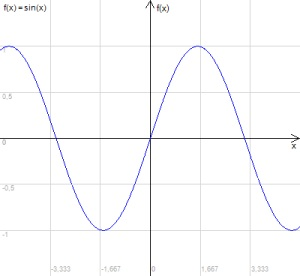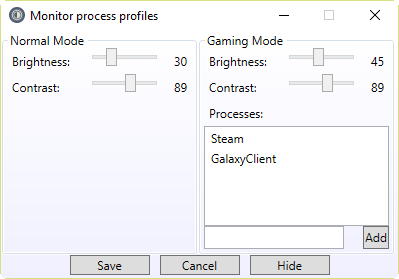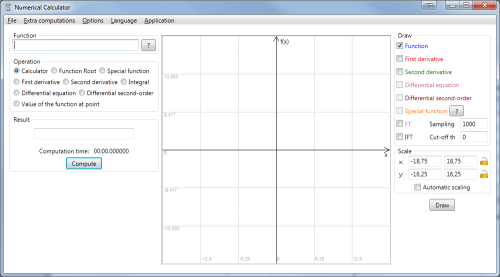### Analytical Interpolation And Approximation .Net Library

Library that returns analytical formula of interpolated or approximated function.

### Chart .Net LibraryLibrary creates function charts from its formulas. You don't need to compute function values just input formula into this library and receive chart of function, its derivative or second derivative.

It can also draw function charts from first and second order differential equations.

Fourier Transform and Inverse FT are supported as well!

### Monitor Process ProfilesApplication allows user to define brightness and contrast of the display for two predefined profiles: Normal Mode and Gaming Mode.

### Numerical CalculatorMain advantage of this program is that you can input complex formulas "as is". No need pressing buttons with functions or numbers. Just write it.

### Numerical Calculator .Net Library

This is easy to use and free numerical .NET library that can compute text math formulas input. This library also include functions able to compute mean and proportion.

### Numerical Derivative .Net Library

This library can compute first and second derivative. Also can be used to compute function value at given point.

### Numerical Differential .Net Library

This library is able to numerically resolve first and second order differential equations.

### Numerical Fourier Transform .Net Library

Fourier Transform library consists of two numerical functions. First one can transform function formula into fourier transform (list of points). The second one is inverse operation that changes list of fourier transform points into list of function value points.

### Numerical Function Root (Hybrid) .Net Library

Library for searching function root at given range.

### Numerical Integral .Net Library

Library for computing integrals.